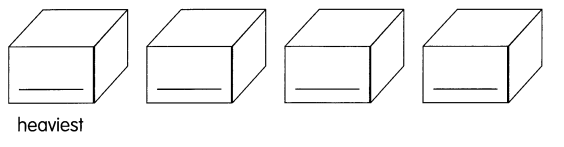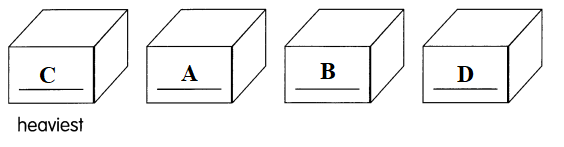# Math in Focus Grade 1 Chapter 10 Practice 3 Answer Key Finding Weight in Units

Practice the problems of Math in Focus Grade 1 Workbook Answer Key Chapter 10 Practice 3 Finding Weight in Units to score better marks in the exam.

## Math in Focus Grade 1 Chapter 10 Practice 3 Answer Key Finding Weight in Units

Fill in the blanks.

Example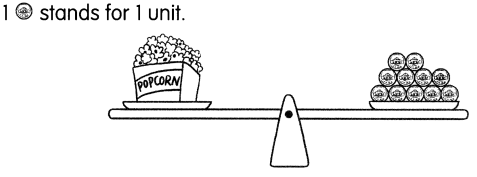The weight of the gift is 11 units.

Question 1.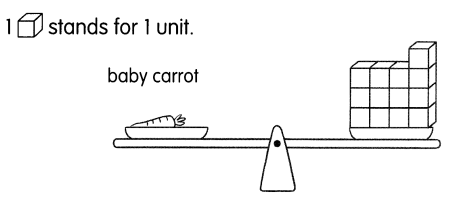The weight of the lemon is ____ units.
Answer: The weight of the baby carrot is 13 units.

Question 2.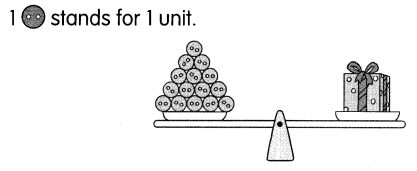The weight of the gift is ____ units.

Question 3.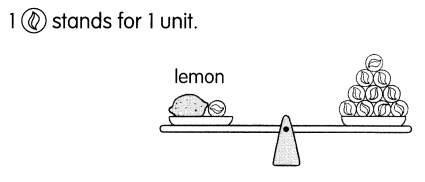The weight of the lemon is ____ units.
Answer: The weight of the lemon is 9 units.

Question 4.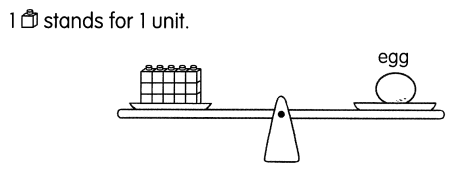The weight of the egg is ____ units.
Answer: The weight of the egg is 15 units.

Question 5.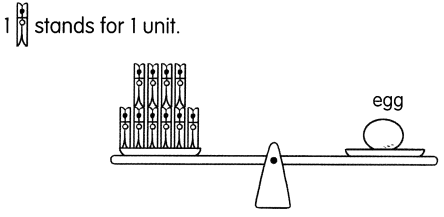The weight of the egg is ___ units.
Answer: The weight of the egg is 10 units.

Fill in the blanks.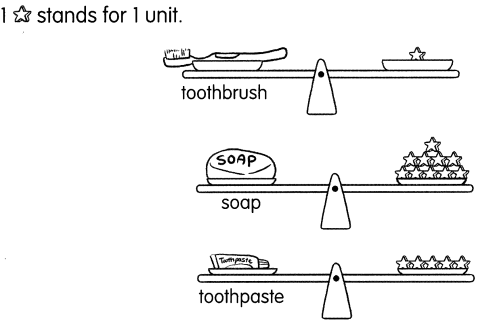Question 6.
The weight of the toothbrush is ____ unit.
Answer: The weight of the toothbrush is 1 unit.

Question 7.
The weight of the soap is ___ units.
Answer: The weight of the soap is 10 units.

Question 8.
The weight of the toothpaste is ___ units.
Answer: The weight of the toothpaste is 5 units.

Question 9.
The ___ is lighter than the toothpaste.
Answer: The toothbrush is lighter than the toothpaste.

Question 10.
The soap is heavier than the ___.
Answer: The soap is heavier than the toothpaste and toothbrush

Question 11.
The ____ is the heaviest.
Answer: The soap is the heaviest.

Question 12.
The ___ is the lightest.
Answer: The toothbrush is the lightest.

Fill in the blanks.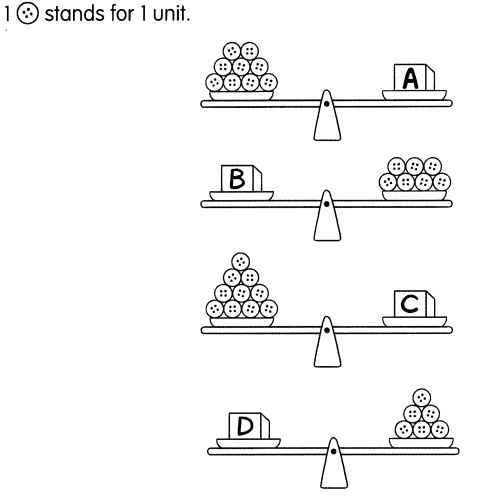Question 13.
The weight of Box A is ____ units.
Answer: The weight of Box A is 9 units.

Question 14.
The weight of Box B is ___ units.
Answer: The weight of Box B is 7 units.

Question 15.
The weight of Box C is ___ units.
Answer: The weight of Box C is 10units.

Question 16.
The weight of Box D is ___ units.
Answer: The weight of Box D is 6 units.

Question 17.
Box ___ is the heaviest.
Answer: Box C is the heaviest.

Question 18.
Box ___ is the lightest.
Answer: Box D is the lightest.

Question 19.
Box ___ is heavier than Box D.
Answer: Box B is heavier than Box D.

Question 20.
Box ___ is lighter than Box A.
Answer: Box B is lighter than Box A.

Question 21.
Arrange the Boxes A to D in order from the heaviest to the lightest.